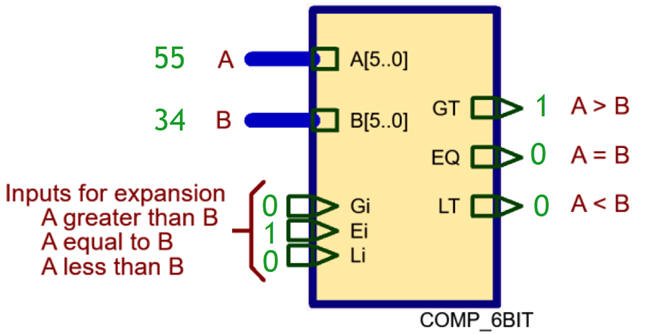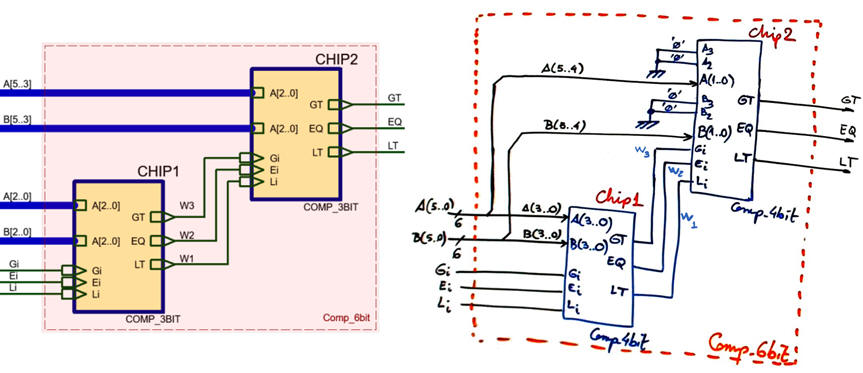﻿ Digital Circuits and Systems - Circuits i Sistemes Digitals (CSD) - EETAC - UPCBachelor's Degree in Telecommunications Systems and in Network EngineeringLecture 2 L3.2: Plan C2 and circuit expandability (circuit scalability) [P3] n-bit arithmetic circuits [14/3]

This tutorial shows how to design an Adder_4bit using Adder_1bit blocks. The key idea in this lecture is discussing the plan C2 internal architecture and study its direct translation to Adder_4bit.vhd (coloured pdf printing; here is explained how to do it):

1.9.1.3. Commercial standard adder chips in classic technologies

This is a commercial standard 4-bit adder  Adder_4bit 74HCT283. It is based on carry lookahead (CLA) equations plan A architecture as shown in tutorial .

1.9.2.2. n-bit comparator (Comp_4bit, Comp_8bit, etc.)

The idea on how to organise a larger comparator, for instance Comp_6bit, is based on using auxiliary inputs Gi, Ei, Li to allow cascading circuits of the same kind.Comp_6bit can be invented using these two approaches shown  below where Comp_4bit or Comp_3bit can be implemented using Comp_1bit:In this way, other comparator tutorial projects can be found: Comp_4bit, Comp_10bit chaining Comp_1bit components.

1.9.2.3. Commercial standard comparator chips in classic technologies

Commercial standard 4-bit comparator Comp_4bit 74F85. It is based on plan A equations as shown in this tutorial .

1.9.3.2. n-bit multipliers

Mult_8bit. This is a Proteus example of hierarchical plan C2 8-bit multiplier Mult_8bit.pdsprj for radix-2 numbers build using a network of expandable 1-bit multiplier cells (Mult_1bit).

Optional. If this circuit had to be designed using VHDL tools, we would choose a behavioural single-file plan B approach so that Quartus Prime will synthesise it efficiently attending the target chip resources. For instance Mult_8bit.vhd / Mult_8bit_tb.vhd. (it is out of the scope of our introductory course and shown only for demonstration purposes).1.9.4. Counting the number of ones in a vector inputs: Ones_counter

1.9.4.1. Ones_counter_4bit

Counting the number of ones in a given data vector:  Ones_counter_4bit. This is the typical application for counting the number of free slots in a parking.

1.9.4.2. Ones_counter_8bit.

This project is highlighted in P3: Ones_counter_8bit.

Indeed, most standard circuits may be inferred in the same way using modular hierarchical structures and repeatable building blocks (plan C2). This is the idea of circuit expandability or scalability proposed as a concept at the mind map. Some examples for both, arithmetic and logic circuit types:

 Circuit using plan C2 Building blocks MUX_8 Mux_2 and MUX_4 Octuple_Mux_4 Mux_2 Dec_4_16 Dec_2_4 Enc_10_4 Enc_4_2 Adder_4bit, Adder_1bit Adder_8bit Adder_1bit, Adder_4bit Comp_6bit Comp_4bit, Comp_1bit Mult_4bit Mult_1bit Ones_counter_8bit Ones_counter_4bit

The same concept will be exploited in Chapter 2 on the design of counters, memories, registers, etc.